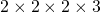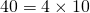# Prime Factors

Grade 10 FMP Introduction to Unit 3

# Factoring integers

To factor an integer or an algebraic expression is to write it as a product. So, 24 = 20+4 is not factoring: here 24 is the result of adding not multiplying so it is not factoring.

Let’s factor the integer 24.24 is a great number for factors because there are so many for such a small number. Here’s another factorization, this time with three factors:What’s the longest line of factors we can write for 24, not including the number 1?This is the longest line. We know its longest because each of the factors in the line are prime – they can’t be broken down into smaller factor pairs.

We say, the prime factorization of 24 isor.

Let’s factor the integer 40.

We know that. We also know thatand that.

So we have.

# Prime Factor Trees

Prime numbers have turned out to be exceptionally useful in our digital world. Any secure websites (emails, bank transactions) use encryption based on prime numbers.

Prime factor trees help to find the prime factorization of any integer.

Here is a how to web page from mathisfun, and a how to video from Khan Academy.

# Practice

Practice drawing prime factor trees. Write a conclusion under each tree, for example,. Usenot a comma in your conclusion.